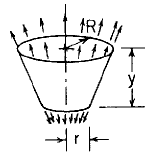Related Resources: pressure-vessel

### Cone Cylinder Tangential loading only Stress and Deflection Equation and Calculator

Cone Cylinder Stress and Deflection by Tangential loading only; resultant load = P. Equation and Calculator. Per. Roarks Formulas for Stress and Strain for membrane stresses and deformations in thin-walled pressure vessels.

 Cone Cylindricalr must be finite to avoid infinite stress and r/t > 10 to be considered thin-walled

Meridional StressCircumferential Hoop StressChange in height dimension yRotation of a meridian from its unloaded positionAngle
α = tan-1 ( ( R-r ) / y)

Where used:
E = Modulus of Elasticity (lbs/in2)
v = Poisson's ratio
P = Applied resultant axial load (lbs)
σ1,2 = stress, (lbs/in2)
α = Angle (deg.)
ψ = Rotation of a meridian from its unloaded position, positive when that meridional rotation represents an increase in ΔR when y or θ increases;

Reference:

Roarks Formulas for Stress and Strain, 7th Edition